# Sum of Products reduction using Karnaugh Map

Boolean Algebra

In this we will learn to reduce Sum of Products (SOP) using Karnaugh Map.

## Reduction rules for SOP using K-map

There are a couple of rules that we use to reduce SOP using K-map first we will cover the rules step by step then we will solve problem. So lets start...

## Pair reduction Rule

Consider the following 4 variables K-map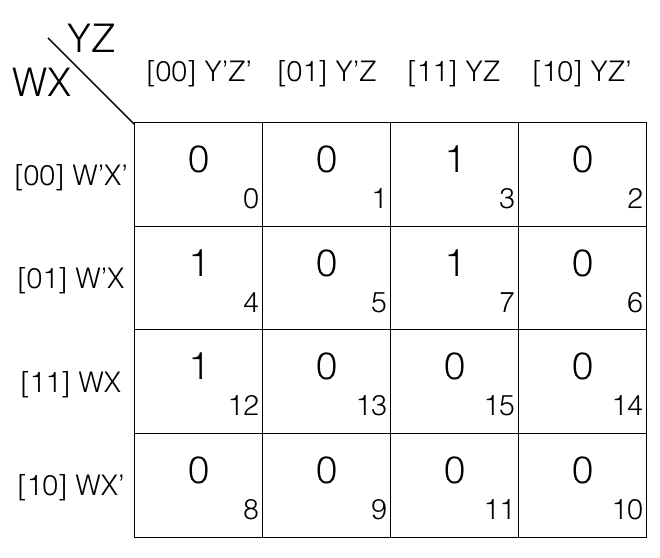Now we mark the cells in pair (set of 2) having value 1.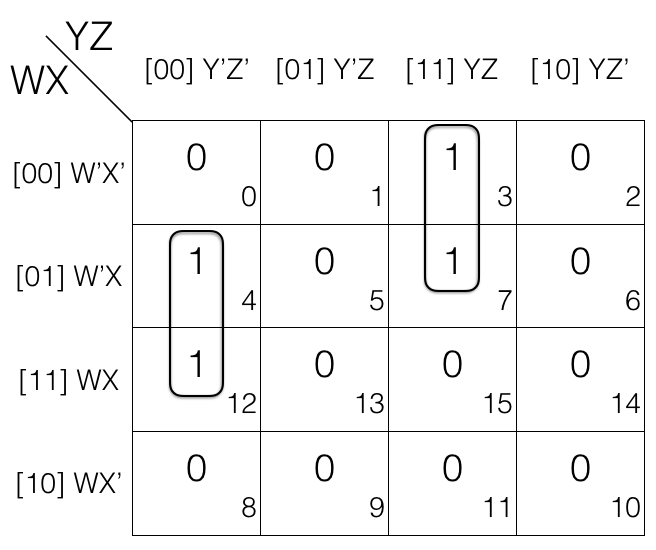The 1st pair = W’XY’Z’ + WXY’Z’
the 2nd pair = W’X’YZ + W’XYZ
(the pairs are in Sum of Products SOP form)

Now we will remove the variable that changed in the 1st and 2nd pair. Looking at the 1st pair W’ changed to W so we remove it. And looking at the 2nd pair X’ changed to X so we remove it.

So the updated pairs after reduction are given below.
1st pair
= W’XY’Z’ + WXY’Z’
= XY’Z’

2nd pair
= W’X’YZ + W’XYZ
= W’YZ

Note!
Pair reduction rule removes 1 variable.

Consider the following 4 variables K-map.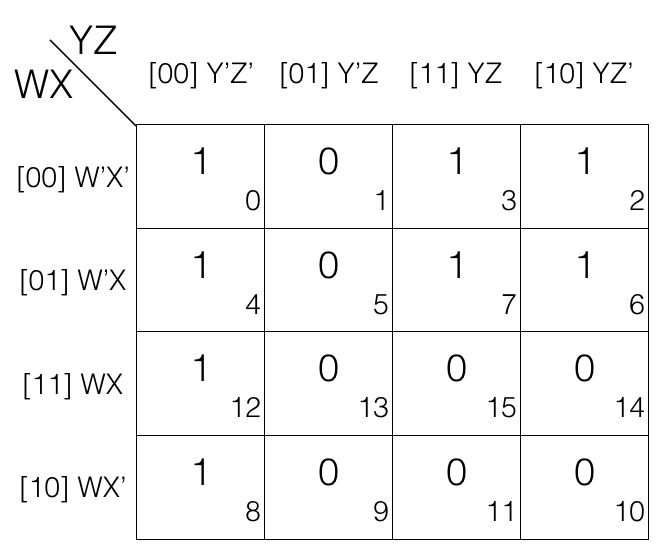Mark the cells in quad (set of 4) having value 1.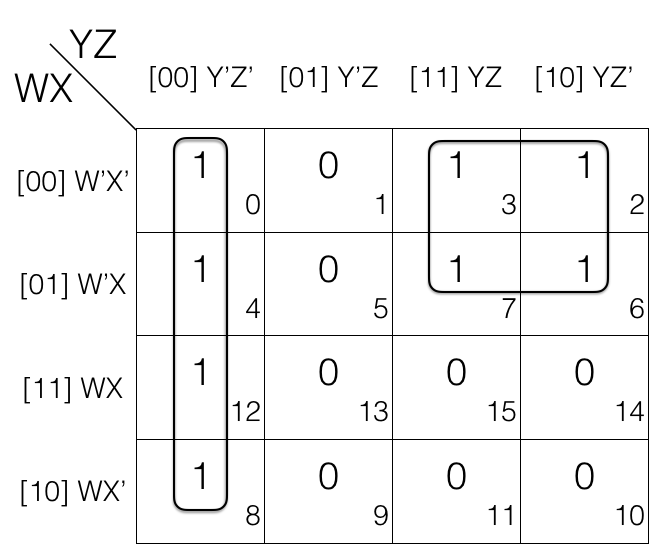The 1st quad = W’X’Y’Z’ + W’XY’Z’ + WXY’Z’ + WX’Y’Z’
The 2nd quad = W’X’YZ + W’XYZ + W’X’YZ’ + W’XYZ’
(the quads are in Sum of Products SOP form)

Now we will remove the variable that changed in the 1st and 2nd quad.

Looking at the 1st quad W’ and X’ changed to W and X so, we remove them. And looking at the 2nd quad X’ and Z changed to X and Z’ so, we remove them.

So the updated quads after reduction.

= (W’X’Y’Z’ + W’XY’Z’) + (WXY’Z’ + WX’Y’Z’)
= W’Y’Z’ + WY’Z’
= Y’Z’

= (W’X’YZ + W’XYZ) + (W’X’YZ’ + W’XYZ’)
= W’YZ + W’YZ’
= W’Y

Note!
quad reduction rule removes 2 variables.

## Octet reduction Rule

Consider the following 4 variables K-map.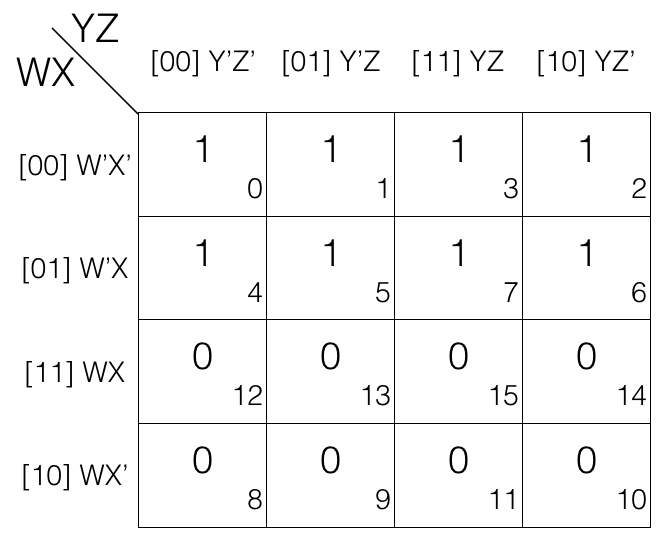Mark the cells in octet (set of 8) having value 1.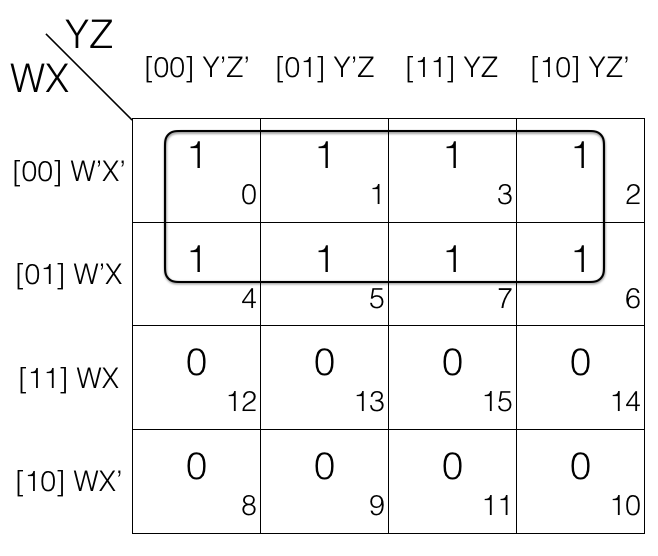octet = W’X’Y’Z’ + W’X’Y’Z + W’X’YZ + W’X’YZ’ + W’XY’Z’ + W’XY’Z + W’XYZ + W’XYZ’
(the octet is in Sum of Products SOP form)

Now we will remove the variable that changed in the octet. Looking at the octet moving top to bottom: X’ changed to X. Moving from left to right: Y’ changed to Y. Moving from left to right: Z’ changed to Z.

Updated octet after reduction
octet
= (W’X’Y’Z’ + W’X’Y’Z) + (W’X’YZ + W’X’YZ’) + (W’XY’Z’ + W’XY’Z) + (W’XYZ + W’XYZ’)
= (W’X’Y’ + W’X’Y) + (W’XY’ + W’XY)
= W’X’ + W’X
= W’

Note!
octet reduction rule will remove 3 variables.

## Map Rolling reduction Rule

In this we consider that the K-map top edge is connected with the bottom edge and left edge is connected with the right edge. Then we mark the pairs, quads and octets. Lets check few examples.

## Map Rolling reduction Rule - marking the pairs

Consider the following 4 variables K-map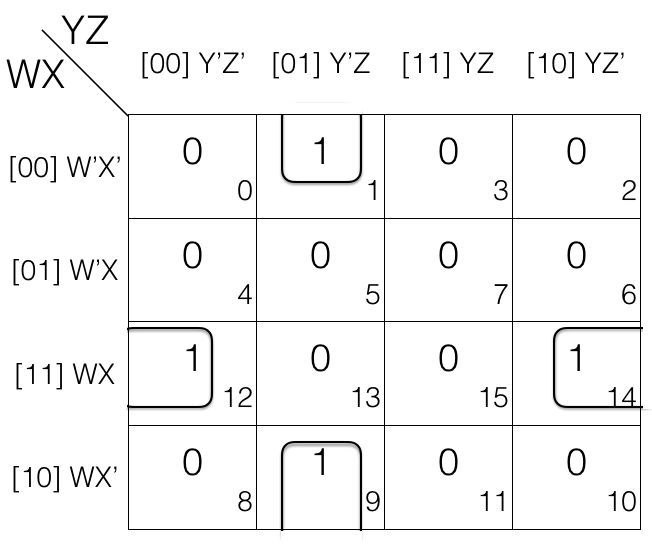In the above k-map we have rolled it and then marked the pairs.

1st pair = W’X’Y’Z + WX’Y’Z
2nd pair = WXY’Z’ + WXYZ’

In 1st pair W’ change to W and in 2nd pair Y’ change to Y. So we will remove them.

Updated pairs after reduction
1st pair
= W’X’Y’Z + WX’Y’Z
= X’Y’Z

2nd pair
= WXY’Z’ + WXYZ’
= WXZ’

## Map Rolling reduction Rule - marking the quads

Consider the following 4 variables K-map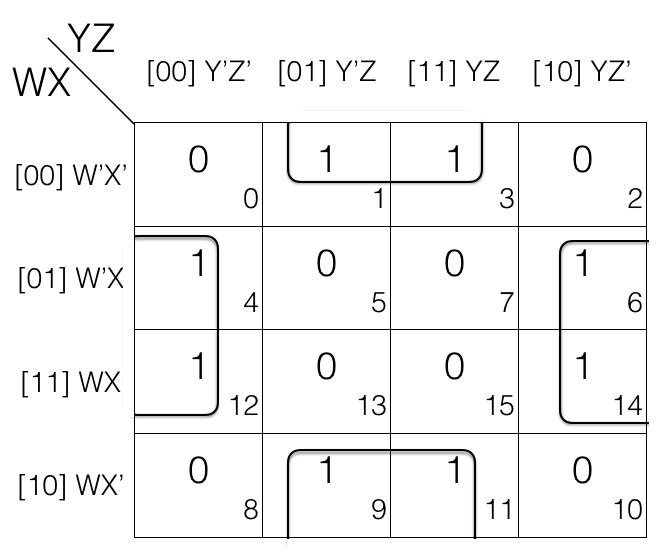In the above k-map we have rolled it and then marked the quads.

= W’X’Y’Z + W’X’YZ + WX’Y’Z + WX’YZ

= W’XY’Z’ + WXY’Z’ + W’XYZ’ + WXYZ’

In 1st quad and 2nd quad W’ change to W and Y’ changes to Y. So we will remove them.

= (W’X’Y’Z + W’X’YZ) + (WX’Y’Z + WX’YZ)
= W’X’Z + WX’Z
= X’Z

= (W’XY’Z’ + WXY’Z’) + (W’XYZ’ + WXYZ’)
= XY’Z’ + XYZ’
= XZ’

## Map Rolling reduction Rule - marking the octets

Consider the following 4 variables K-map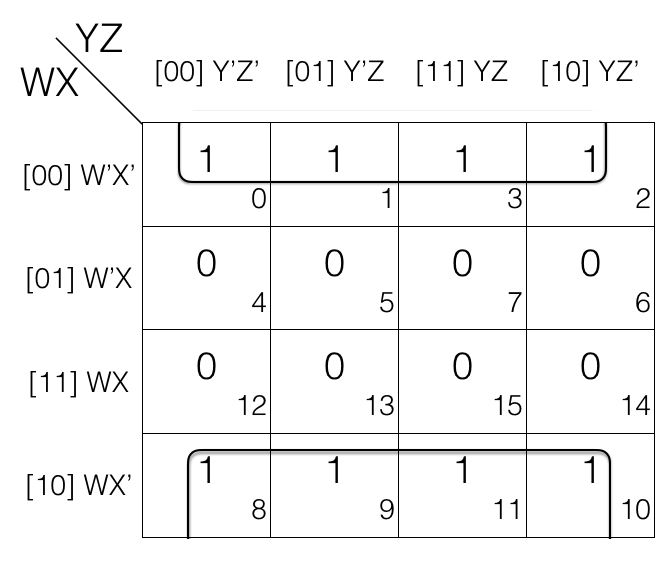In the above k-map we have rolled it and then marked the octet.

octet
= W’X’Y’Z’ + W’X’Y’Z + W’X’YZ + W’X’YZ’ + WX’Y’Z’ + WX’Y’Z + WX’YZ + WX’YZ’

Looking at the octet we can tell that W’ changed to W, Y’ changed to Y and Z’ changed to Z. So we will remove them.

Updated octet after reduction

octet
= (W’X’Y’Z’ + W’X’Y’Z) + (W’X’YZ + W’X’YZ’) + (WX’Y’Z’ + WX’Y’Z) + (WX’YZ + WX’YZ’)
= (W’X’Y’ + W’X’Y) + (WX’Y’ + WX’Y)
= W’X’ + WX’
= X’

## Overlapping Groups

When a value in a cell of K-map is encircled in more that one group (pair, quad or octet) then we call such groups an overlapping groups. Lets check an example.

Consider the following 4 variables K-map.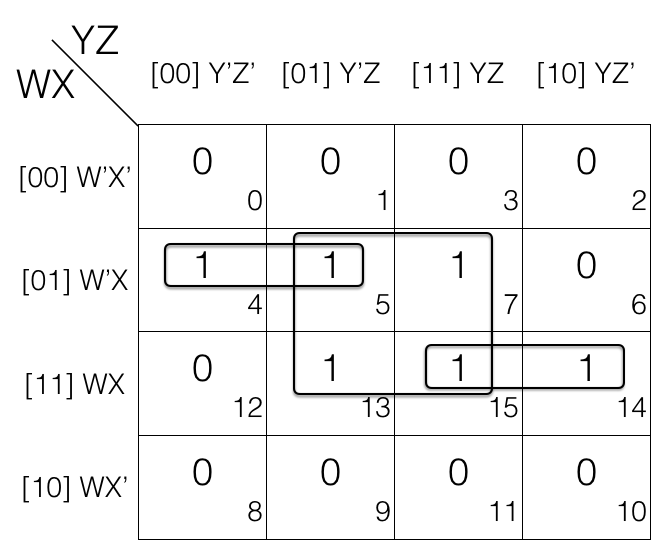1st pair = W’XY’Z’ + W’XY’Z
= m4 + m5

2nd pair = WXYZ + WXYZ’
= m15 + m14

quad = W’XY’Z + W’XYZ + WXY’Z + WXYZ
= m5 + m7 + m13 + m15

If we look at m5 and m15, they are overlapping.

Overlapping groups helps in getting simpler expression after reduction.

Updated pair and quad after reduction

1st pair = W’XY’Z’ + W’XY’Z
= W’XY’

2nd pair = WXYZ + WXYZ’
= WXY

quad = W’XY’Z + W’XYZ + WXY’Z + WXYZ
= W’XZ + WXZ
= XZ

## Redundant Groups

After marking out the overlapping groups it is important to also check for redundant groups. If all the values of a group G (pair, quad or octet) is covered (overlapping) with other groups then that group G is redundant and ignored. Lets check an example.

Consider the following 4 variables K-map.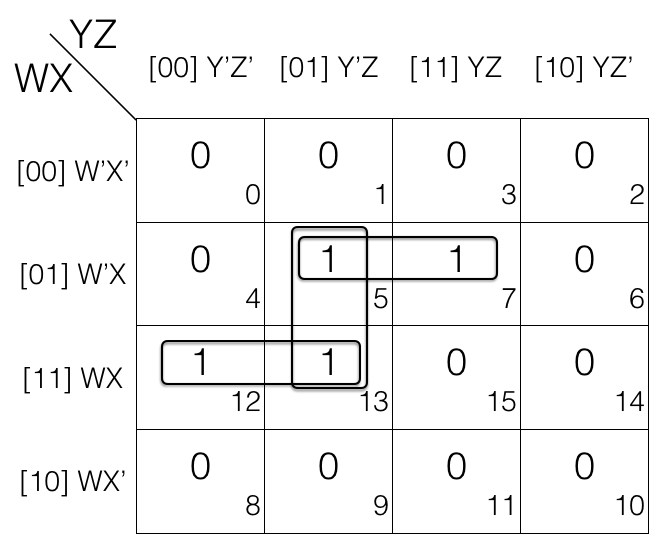1st pair = W’XY’Z + W’XYZ = m5 + m7

2nd pair = WXY’Z’ + WXY’Z = m12 + m13

3rd pair = W’XY’Z + WXY’Z = m5 + m13

If we look at m5 and m13 i.e. 3rd pair, it is a redundant group as m5 and m13 is covered in 1st and 2nd pair so we will remove the redundant group (3rd pair).

Updated pairs after reduction

1st pair = W’XY’Z + W’XYZ
= W’XZ

2nd pair = WXY’Z’ + WXY’Z
= WXY’

## Summary of Reduction rules for SOP using K-map

1. Prepare the truth table for the function
2. Draw an empty K-map (2-variables, 3-variables, so on)
3. Fill the cells with value 1 for which the output is 1
4. Fill rest of the cells with value 0
5. Mark the Octets, Quads and Pairs by encircling the value 1s (also check map rolling, overlapping groups and remove redundant groups)
6. Write the final reduced expression and OR (+) them to get the answer

Now let us solve a problem.

## Reduce F(A,B,C,D) = ∑(0,1,2,4,5,7,10,15) using K-map

Since function F has 4 variables so we will create a 4 variable K-map having 24 = 16 cells.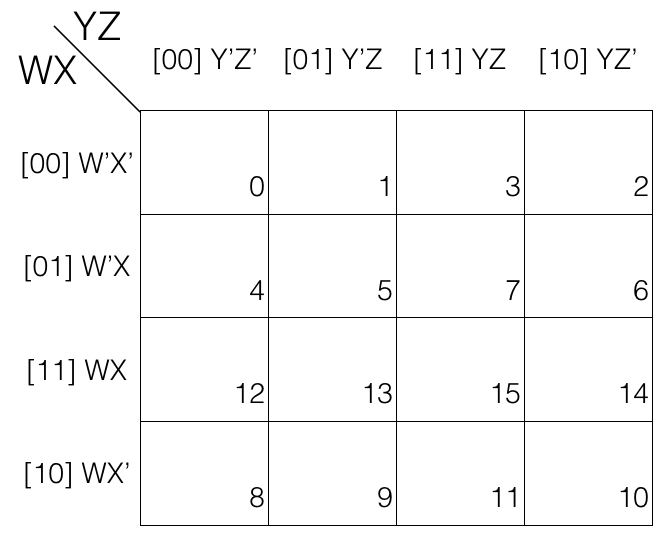Now fill the cell marked with subscript 0,1,2,4,5,7,10 and 15 with value 1 as we are dealing with Sum of Products SOP.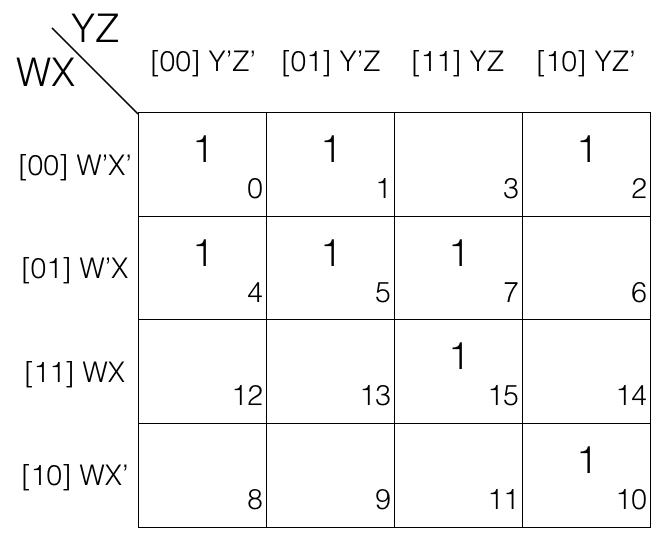And fill rest of the cells with value 0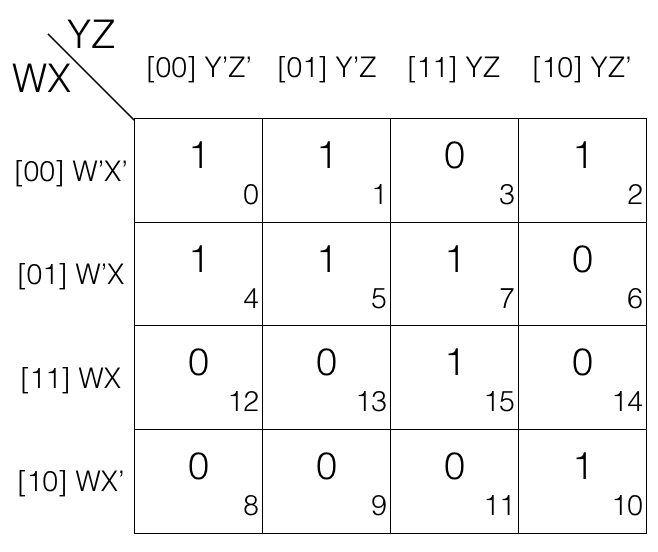Now we will mark the octets, quads and pairs.

Looking at the K-map we can tell that there is no octets so we will look for quads.

The k-map has quad so we will mark it.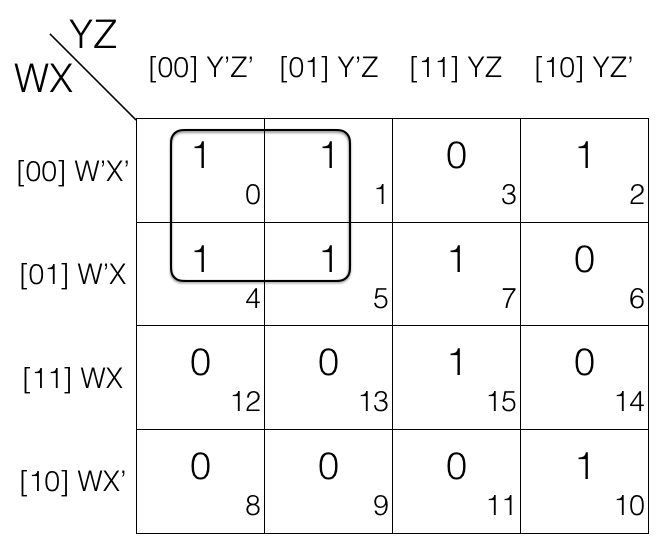Now we will look for pairs. If we look close we can tell that the k-map has some pairs. So we will mark them.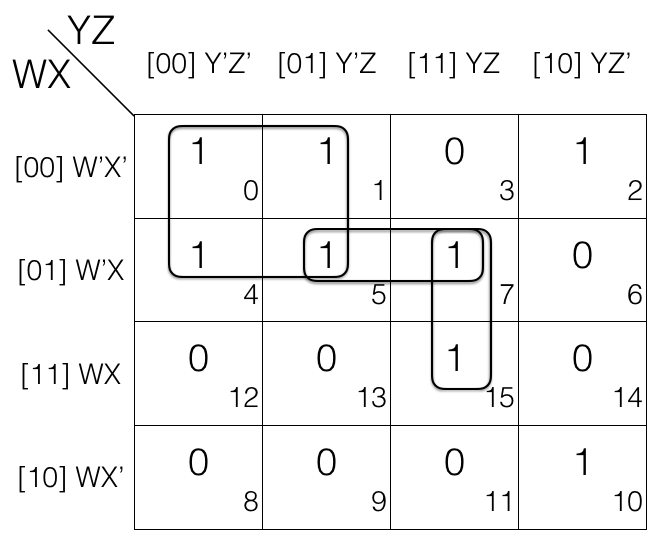Now we will map-roll and look for octets. If we look at the k-map we can tell that after map rolling we don't get any octet. So we will now check for quads.

We roll the k-map again and this time we are looking for quads. But giving a closer look we can tell that there is no quad after we do map rolling. So time for us to look for pairs.

This time we are lucky. Looking closer we can tell that after map rolling the k-map we have a pair. So we will mark it.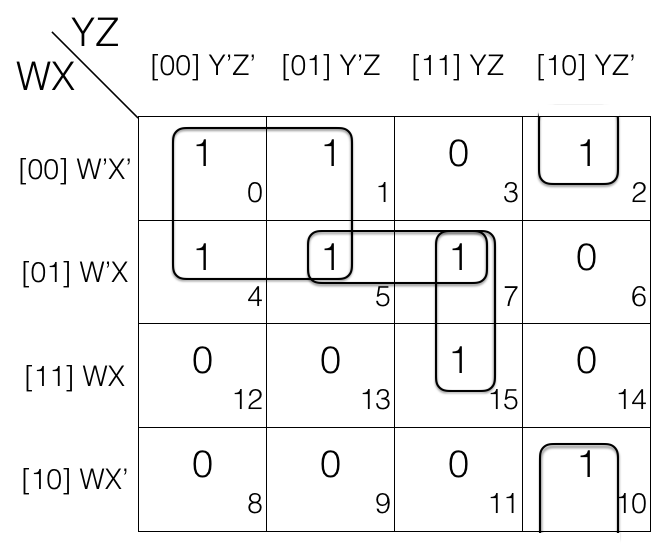Now we will look for overlapping groups.

If we look at the K-map we will see that m7 in pair (m5, m7) and pair (m7, m15) is shared in both the groups. So both the pairs are overlapping groups.

Similarly m5 in pair (m5, m7) and quad (m0, m1, m4, m5) is shared in both the groups. So the pair and quad are overlapping groups.

And there is no other overlapping groups so, we will now check for redundant groups.

Looking at the K-map we can tell pair (m5, m7) is redundant as m5 is covered in quad (m0, m1, m4, m5) and m7 is covered in pair (m7, m15). So we will remove the pair (m5, m7).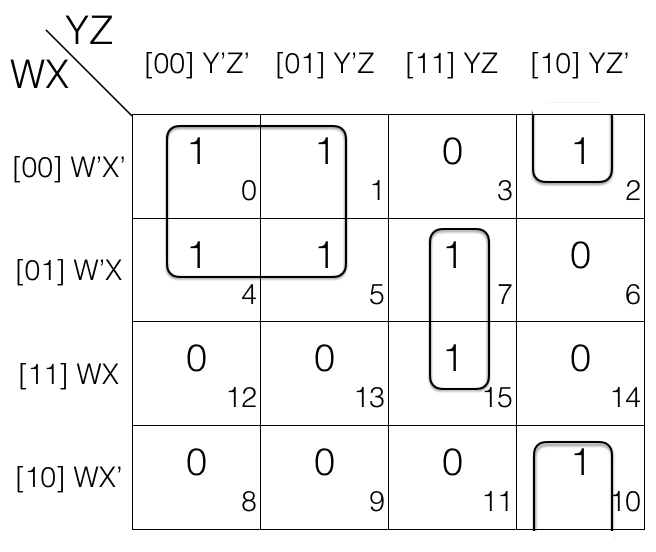Now we will write down the marked groups and find the reduced expression.

quad = (m0, m1, m4, m5) = (W’X’Y’Z’ + W’X’Y’Z) + (W’XY’Z’ + W’XY’Z)
= W’X’Y’ + W’XY’ [Z’ changed, so removed]
= W’Y’ [X’ changed to X, so removed]

1st pair = (m7, m15)
= W’XYZ + WXYZ
= XYZ [W’ changed to W, so removed]

2nd pair = (m2, m10)
= W’X’YZ’ + WX’YZ’
= X’YZ’ [W’ changed to W, so removed]

Now we OR (+) the results to get the final reduced expression.

F = W’Y’ + XYZ + X’YZ’